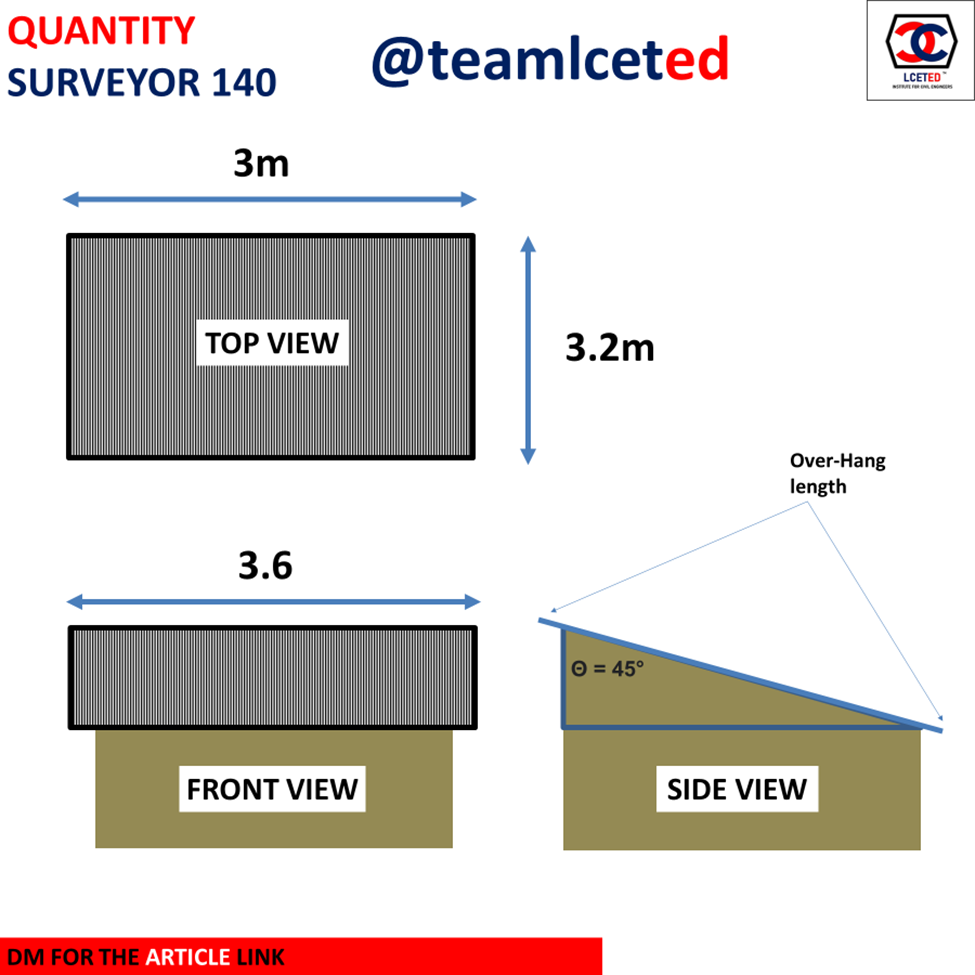Roof Sheet Calculator | How to calculate Number Roof Sheets Required? -lceted LCETED INSTITUTE FOR CIVIL ENGINEERS

## Nov 14, 2022

In this Article, We explained How to calculate Roof sheets for Single Slope (Skillion) Roof. and also we have attached the Roof sheet excel calculator for your use.Step 1: Take Roof Measurements

Note: Consider the Length of the Roof Is water flow directions, For Example

Example

Width of Roof = 3m

Length (water flow direction) = 3.2m

Roof Angle = 45°

Step 2: Calculate Roof Length and width For Sheet Calculation

A. Roof Width

Width of Roof = Width of (outer wall to wall) + (2 x overhang sheet length)

Consider Overhang length = 0.3m

Width of Roof = 3 + (2 x 0.3) = 3.6m

Length of Roof (water flow direction) = Length + (2 x overhang sheet length)

Length of Roof (water flow direction), As we know Water flow direction will be sloped. So, we need to find Sloping Length of the roof

Length of Roof (water flow direction) =

As per Trigonometry, sin θ = opposite / hypotenuse

Consider this Equation on Above Triangle,

We will take,

Side (AC) (water flow direction) = Hypotenuse =?

Side (BC) = Opposite = 3.2m

So,

As we know Roof Angle θ = 45°

Sin45° = (3.2m/hypotenuse)

0.707= (3.2/hypotenuse)

By Cross multiplying,

Hypotenuse = 3.2/ 0.707 = 4.526m

So, the length (water flow direction) of the Roof 4.526m

Length of Roof = Length + (2 x overhang sheet length)

Length of Roof = 4.526 + 0.6 = 5.126m

Step 3: Roof Sheet Calculation

Consider Roof Sheet Size

Length (water flow direction) of Roof Sheet = 2.4m

Width of Roof sheet = 1.2m

The above image gives you an idea of the Overlapping Area

Number of Sheet Needed in one Row of Roof width = Width of Roof/Width of Roof Sheet = 3.6/1.2 = 3nos

Overlapping = Number of sheets -1 = 3-1 = 2 nos

Consider 300mm as the overlapping length so,

Overlapping = 2 x 300 = 600mm = 0.6m

Total Width of Roof = 3.6 + overlap length = 3.6+0.6 = 4.2m

Number of Sheets Needed (overlapping) in width side = 4.2/1.2 = 3.5 – 4 sheets

Number of Sheet Needed in one Row of Roof length (water flow direction) = Length of Roof (water flow direction) / Length of Roof Sheet = 5.126/2.4 = 2.135 no’s

Overlapping = Number of sheets -1 = 2.135-1 = 1.135 - 1 nos

Consider 300mm as the overlapping length so,

Overlapping = 1 x 300 = 300mm = 0.3 m

Total length of Roof = 5.126 + overlap length = 5.126+0.3 = 5.426m

Number of Sheets Needed (overlapping) in Length side = 5.426/2.4 = 2.26 – 2.5

Total Number of Sheets Needed = 4 (Width) x 2.5 (Length) = 10 sheets

Below We have Attached the Roof sheet calculator for the Single slope Roof For your use.